# Difference: DataFitting (4 vs. 5)

Line: 1 to 1

 META TOPICPARENT name="SubmitCaseStudy"
<-- Under Construction -->
Line: 26 to 26

Changed:
<
<

>
>

## Problem Description

When modelling data, scientists often want to fit an analytical model to their experimental data. In this case an experiment has provided some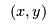-coordinates that have a growth curve shape, i.e., initially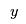increases quickly with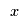, thens tails off to some maximum value. The scientists in this study have suggested three possible functions to model this behaviour:

1.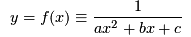2.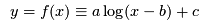3.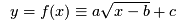Given one of the possible functions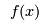and the data points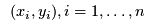they want to find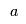,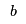and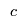to minimize the squared distance between the data points and the estimates, i.e.,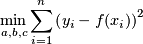The scientists have specified the number of data points and their coordinates in an AMPL data file regression.dat.

The scientists want to know which of the suggested functions provides the best fit to the data.

Changed:
<
<

>
>

## Problem Formulation

Changed:
<
<
The formulation...
>
>
The formulation...

Changed:
<
<

## Computational Model

>
>

Changed:
<
<
The computational model...
>
>

Changed:
<
<

## Results

>
>
The computational model...

Changed:
<
<
The results...
>
>

Changed:
<
<

>
>

Changed:
<
<
In conclusion...
>
>
The results...

Changed:
<
<
>
>

## Conclusions

In conclusion...

Changed:
<
<
>
>

Changed:
<
<
>
>

Changed:
<
<
>
>

Changed:
<
<

>
>

Changed:
<
<
1. Write AMPL files regression.mod and regression.run that use regression.dat to solve The Data Fitting Problem. Write a management summary of your solution. Be sure to indicate which of the functions fits the data best.

What to hand in Your new AMPL files regression.mod and regression.run. Your management summary.

>
>

Changed:
<
<
>
>

 META FORM FORM FIELD Title name="OpsRes.CaseStudyForm" Title DataFitting DateSubmitted 19 Feb 2008
>
>
 FORM FIELD CaseStudyType CaseStudyType DIYCaseStudy

 FORM FIELD OperationsResearchTopics FORM FIELD ApplicationAreas OperationsResearchTopics NonlinearProgramming ApplicationAreas Data Analysis
|*FORM FIELD ProblemDescription*|ProblemDescription|*THE DATA FITTING PROBLEM*

Also known as The Least Squares Problem

When modelling data, scientists often want to fit an analytical model to their experimental data. In this case an experiment has provided some-coordinates that have a growth curve shape, i.e., initiallyincreases quickly with, thens tails off to some maximum value. The scientists in this study have suggested three possible functions to model this behaviour:

1.2.3.Given one of the possible functionsand the data pointsthey want to find,andto minimize the squared distance between the data points and the estimates, i.e.,The scientists have specified the number of data points and their coordinates in an AMPL ??? LINK data file =regression.dat=.

The scientists want to know which of the suggested functions provides the best fit to the data.|

Copyright © 2008-2021 by the contributing authors. All material on this collaboration platform is the property of the contributing authors.
Ideas, requests, problems regarding TWiki? Send feedback